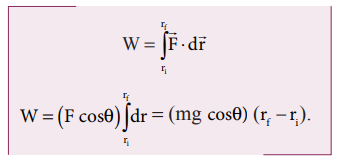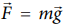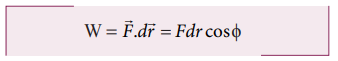Home | | Physics 11th std | Work done by a constant force

# Work done by a constant force

When a constant force F acts on a body, the small work done (dW) by the force in producing a small displacement dr.

Work done by a constant force

When a constant force F acts on a body, the small work done (dW) by the force in producing a small displacement dr is given by the relation,The total work done in producing a displacement from initial position ri to final position rf is,The graphical representation of the work done by a constant force is shown in Figure 4.5. The area under the graph shows the work done by the constant force.The component mg cosθ and the normal force N are perpendicular to the direction of motion of the object, so they do not perform any work.

## Solved Example Problems for Work

### Example 4.1

A box is pulled with a force of 25 N to produce a displacement of 15 m. If the angle between the force and displacement is 30o, find the work done by the force.### Solution

Force, F = 25 N

Displacement, dr = 15 m

Angle between F and dr, θ = 30o

Work done, W = Fdr cosθ## Solved Example Problems for Work done by a constant force

Example 4.2

An object of mass 2 kg falls from a height of 5 m to the ground. What is the work done by the gravitational force on the object? (Neglect air resistance; Take g = 10 m s-2)### Solution

In this case the force acting on the object is downward gravitational force. This is a constant force.

Work done by gravitational force isThe object also moves downward which is in the direction of gravitational force () as shown in figure. Hence, the angle between them is θ = 0° cos0° = 1 and the displacement, (rf - r1)  = 5mThe work done by the gravitational force on the object is positive.

Example 4.3

An object of mass m=1 kg is sliding from top to bottom in the frictionless inclined plane of inclination angle θ = 30o and the length of inclined plane is 10 m as shown in the figure. Calculate the work done by gravitational force and normal force on the object. Assume acceleration due to gravity, g = 10 m s-2### Solution

We calculated in the previous chapter that the acceleration experienced by the object in the inclined plane as g sinθ .

According to Newton’s second law, the force acting on the mass along the inclined plane F = mg sinθ. Note that this force is constant throughout the motion of the mass.

The work done by the parallel component of gravitational force ( mg sinθ ) is given bywhere ϕ is the angle between the force (mg sin θ) and the direction of motion (dr). In this case, force (mg sin θ) and the displacement () are in the same direction. Hence ϕ = o and cos ϕ = 1### Example 4.4

If an object of mass 2 kg is thrown up from the ground reaches a height of 5 m and falls back to the Earth (neglect the air resistance). Calculate

a) The work done by gravity when the object reaches 5 m height

b) The work done by gravity when the  object comes back to Earth

c) Total work done by gravity both in upward and downward motion and mention the physical significance of the result.

### Solution

When the object goes up, the displacement points in the upward direction whereas the gravitational force acting on the object points in downward direction. Therefore, the angle between gravitational force and displacement of the object is 180°.

a. The work done by gravitational force in the upward motion.

Given that ∆r =5 m and F mgb. When the object falls back, both the gravitational force and displacement of the object are in the same direction. This implies that the angle between gravitational force and displacement of the object is 0°.c. The total work done by gravity in the entire trip (upward and downward motion)It implies that the gravity does not transfer any energy to the object. When the object is thrown upwards, the energy is transferred to the object by the external agency, which means that the object gains some energy. As soon as it comes back and hits the Earth, the energy gained by the object is transferred to the surface of the Earth (i.e., dissipated to the Earth).

### Example 4.5

A weight lifter lifts a mass of 250 kg with a force 5000 N to the height of 5 m.

a. What is the workdone by the weight lifter?

b. What is the workdone by the gravity?

c. What is the net workdone on the object?

### Solution

a. When the weight lifter lifts the mass, force and displacement are in the same direction, which means that the angle between them θ = 00. Therefore, the work done by the weight lifter,b. When the weight lifter lifts the mass, the gravity acts downwards which means that the force and displacement are in opposite direction. Therefore, the angle between them θ = 1800c. The net workdone (or total work done) on the objectStudy Material, Lecturing Notes, Assignment, Reference, Wiki description explanation, brief detail
11th Physics : UNIT 4 : Work, Energy and Power : Work done by a constant force |